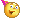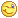We have been working very hard since 2009 to facilitate in your learning Read More. We can't keep up without your support. Donate Now.

#www.vustudents.ning.com

 www.bit.ly/vucodes + Link For Assignments, GDBs & Online Quizzes Solution www.bit.ly/papersvu + Link For Past Papers, Solved MCQs, Short Notes & MoreLooking For Something at Site? Search Below

# CS606 Compiler Construction Assignment No 02 Solution & Discussion Due Date:16-05-2013

a) Show that the grammar
is ambiguous by finding a string that has two different syntax trees.
Now make two different unambiguous grammars for the same language:
a) One where prefix minus binds stronger than infix minus.
b) One where infix minus binds stronger than prefix minus.
Show the syntax trees using the new grammars for the string you used to prove
the original grammar ambiguous.
Question No 2: Marks 10
Consider the grammar
A → B C D
B → h B | ε
C → C g | g | C h | i
D → A B |ε
Fill-in the table below with the sets First and Follow. Remember to put ε in the set First(X)
whenever X can reduce to the empty string.
First Follow
A
B
C
D

+ http://bit.ly/vucodes (Link for Assignments, GDBs & Online Quizzes Solution)

+ http://bit.ly/papersvu (Link for Past Papers, Solved MCQs, Short Notes & More)

Views: 1773

Attachments:

### Replies to This Discussion

friends iss ka solution jaldi upload kr dejiye ga

idia

Attachments:

Our main purpose here discussion not just Solution

We are here with you hands in hands to facilitate your learning and do not appreciate the idea of copying or replicating solutions.

plz solution ms office 2003 mein snd kia kren

Idea Solution

Problem No.1

Find the First and Follow sets for the grammar

S ® ABC

A ® a | Cb | e

B ® c | dA | e

C ® e | f

Solution:

First:

First(S) = First(ABC) = { a, c, d, e, f}

First(A)                      = { a, e, f, e }

First(B)                     = {c, d, e }

First(C)  = First(CB)  = {e, f}

Follow:

Follow(S) = {\$}

Follow(A) = {c, d, e, f }

Follow(B) = {e, f}

Follow(C) = {b, \$}

Problem No.2

Find the First and Follow sets for the grammar

Grammar is give

(I am using or instead of usual | to avoid confusion)

a) Find the First and Follow sets for the grammar

E  ® TP

P ® e or |TP

T ® FQ

Q ® e or FQ

F ® (E) R or iR

R ® e or *R

Solution:

First(E)  = { (, i}

First(P) = { e, | }

First(T) = { (, i}

First(Q) = { (, i, e}

First(F) = { (, i}

First(R) = { *, e}

Follow(E)   = { \$, )}

Follow (P)  = { \$, ) }

Follow (T)  = {\$, ), |}

Follow (Q) = { \$, ),| }

Follow (F)  = { \$,(, ), i, |}

Follow (R) = { \$, (, ), i, |}

b) Construct LL(1) parsing tables from grammar

Solution:

LL(1) parsing table:

 | ( ) i * \$ E TP TP P |TQ e e T FQ FQ Q e FQ e FQ e F (E)R iR R e e e e *R e

CS606 kisi ka ni kia,

kahan ho sb log, koi ni CS606 wala shehzada

Dear Students Share your views and problems about this Assignment here, So we can discuss and solve this Assignment, Don't wait for solutions , Start discussion because Discussion clear our concepts & provide best solutions.

plz help me any one

koi is ko b discuss kr lyYar table me kiya values put karni hain discuss about it . first Question me to tree banay ga just. What will be the values whom we need to put in table. 1st easy hai 2nd k baray me discuss krein plxx.Mr Ali first i think sarif tree  ahi banay bilka grammer ko phala unambiguous grammer ma convert karna ha phir us ka tree banay ga

plz tell am i right

check these

Frist(A)={h,g,i,epsilon}

Frist(B)={h,epsilon}

Frist(C)={g,i}

Frist(D)={g,h,i,epsilon}

.